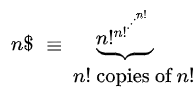# Superfactorial: Definition (Sloane, Pickover’s)

The term superfactorial has two slightly different definitions: as a product of factorials (Sloane & Plouffe, 1995) or as a tower of factorials involving compound exponents (Pickover, 1995). Sloan and Pouffe’s form is the most common.

## 1. Sloane & Plouffe’s Superfactorial

A superfactorial is defined by Sloane and Plouffe as the product of the first n factorials. Their definition is equivalent to the integral values for the Barnes G-function.

## Examples

The superfactorial of 3 is:
sf(3) = 1 factorial * 2 factorial * 3 factorial = 1 * 2 * 6 = 12

Superfactorials for integers 1 through 11 are (OEIS A000178):

1. 1
2. 2
3. 12
4. 288
5. 34560
6. 24883200
7. 125411328000
8. 5056584744960000
9. 1834933472251084800000
10. 6658606584104736522240000000
11. 265790267296391946810949632000000000
12. 127313963299399416749559771247411200000000000

## Pickover’s Superfactorial

Pickover (1995) defines a different superfactorial, one that involves compound exponentiation:The dollar sign (\$) is actually a factorial symbol (an exclamation mark !) overwritten with the letter S (Mudunuru et al., 2017).
This can also be expressed as a tetration:
n\$ = n! (n!).

Alternatively, it can be expressed as a tower of exponents, using Knuth’s arrow up notation:
So a ↑↑n, is iterated exponentiation (i.e.tetration), and means to raise a to itself n – 1 times. For example,

a ↑↑5 = aaaaa.

## Examples

The first two values are:

• 1\$ = 1,
• 2\$ = 3

From n = 3 this grows very rapidly and up the numbers are huge. \$n is roughly 10101036305.

## References

Fletcher, A.; Miller, J. C. P.; Rosenhead, L.; and Comrie, L. J. An Index of Mathematical Tables, Vol. 1. Oxford, England: Blackwell, p. 50, 1962.
Graham, R. L.; Knuth, D. E.; and Patashnik, O. Concrete Mathematics: A Foundation for Computer Science, 2nd ed. Reading, MA: Addison-Wesley, p. 231 1994.
Mudunuru et al. Zero Factorial. Sch. J. Phys. Math. Stat. 2017; 4(4):172-177
Pickover, C. A. Keys to Infinity. New York: Wiley, p. 102, 1995.
Ryser, H. J. Combinatorial Mathematics. Buffalo, NY: Math. Assoc. Amer., p. 53, 1963.
Sloane, Plouffe. The Encyclopedia of Integer Sequences. Academic Press, 1995

CITE THIS AS:
Stephanie Glen. "Superfactorial: Definition (Sloane, Pickover’s)" From StatisticsHowTo.com: Elementary Statistics for the rest of us! https://www.statisticshowto.com/superfactorial-definition-sloane-pickovers/
---------------------------------------------------------------------------Need help with a homework or test question? With Chegg Study, you can get step-by-step solutions to your questions from an expert in the field. Your first 30 minutes with a Chegg tutor is free!